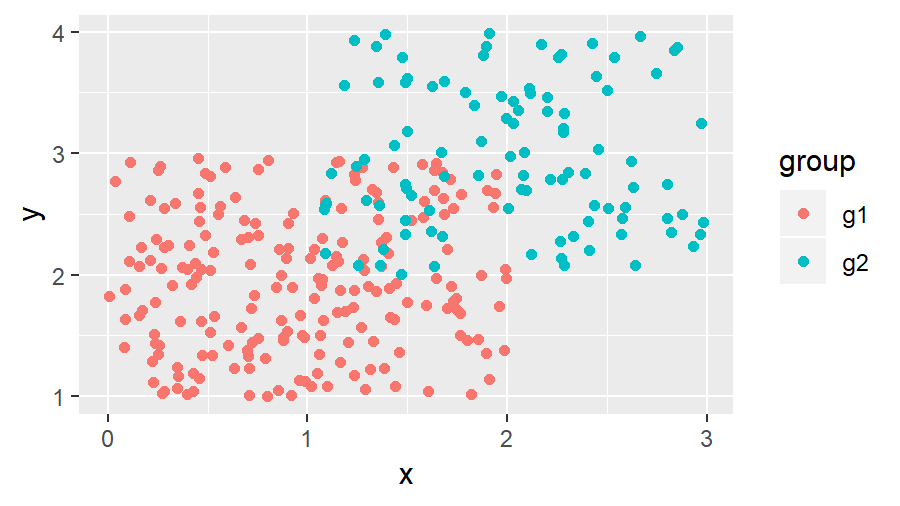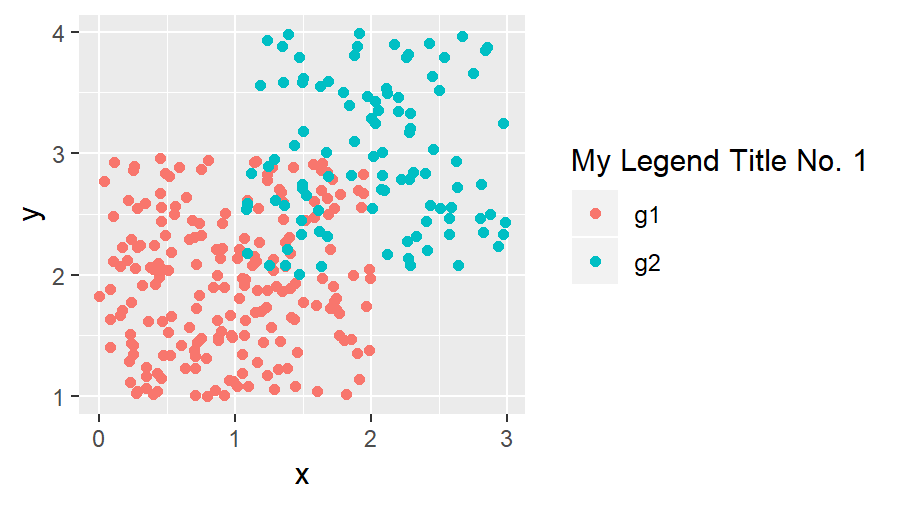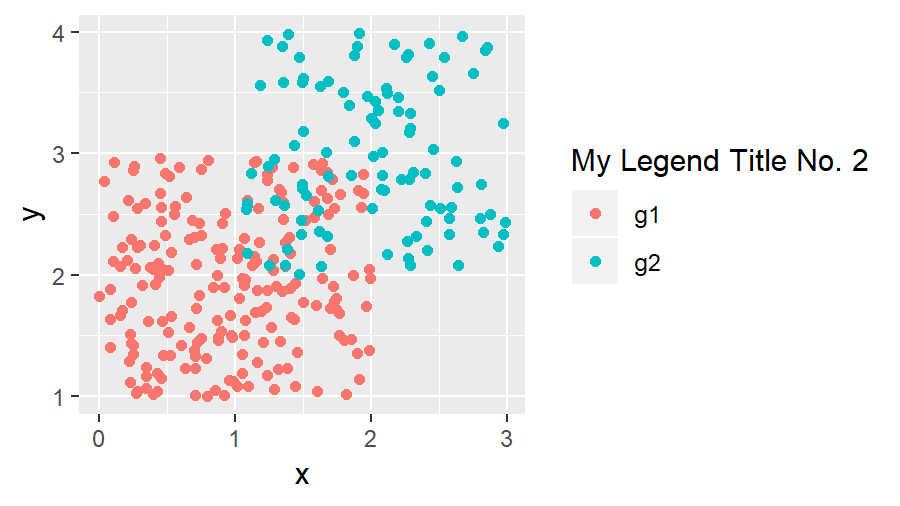# Change Legend Title in ggplot2 (2 Example Codes) | Modify Text of ggplot Legends

This article explains how to change the text of a ggplot2 legend in R. The tutorial is structured as follows:

Let’s move on to the examples!

## Create Example Data

First, we have to create some data for the examples of this tutorial:

```set.seed(2468) # Set seed for reproducibility data <- data.frame(x = c(runif(200, 0, 2), runif(100, 1, 3)), # Create x variable y = c(runif(200, 1, 3), runif(100, 2, 4)), # Create y variable group = c(rep("g1", 200), rep("g2", 100))) # Create group variable head(data) # Print first 6 rows of data # x y group # 1 0.9251444 2.510920 g1 # 2 1.1482466 1.688871 g1 # 3 0.7495806 1.476128 g1 # 4 1.8013962 1.462917 g1 # 5 0.4498683 2.673079 g1 # 6 0.6840810 2.449923 g1```

Our example data frame contains an x variable, a y variable, as well as a group variable.

Now let’s see how the legend title looks by default. First, we need to install and load the ggplot2 package in R…

```install.packages("ggplot2") # Install ggplot2 package library("ggplot2") # Load ggplot2 package```

…and then we can print a ggplot2 scatterplot as follows:

```my_ggplot <- ggplot(data, aes(x, y, group = group)) + # ggplot2 with 2 legends geom_point(aes(x, y, color = group)) my_ggplot # Print plot with default legend```Figure 1: ggplot2 with Default Specification.

As you can see based on Figure 1, the default specification of the ggplot2 package shows the column name of our group variable as legend title.

In the following examples, I’ll show you two alternatives how to change the text of this legend title in R.

Let’s dive in!

## Example 1: Change Text of ggplot Legend Title with scale_color_discrete

This example shows how to modify a ggplot legend title with scale_color_discrete:

`my_ggplot + scale_color_discrete(name = "My Legend Title No. 1") # Manual legend title`Figure 2: ggplot2 with Legend Title Modified by scale_color_discrete.

The previous R syntax changed the title to “My Legend Title No. 1”.

Note: We used the function scale_color_discrete, because our legend is created based on the color specification of our graphic. If we would have created our legend based on other aesthetics, we would have to use the corresponding scale_…_discrete function (e.g. scale_fill_discrete for fill). This is by the way true for all types of plots (e.g. histogram, barchart, dotplot, pairs plot and so on).

There’s another alternative, which is also quite popular for changing legend titles of ggplots. So keep on reading…

## Example 2: Change Text of ggplot Legend Title with labs

The second alternative that I want to show you is based on the labs function:

`my_ggplot + labs(color = "My Legend Title No. 2") # Manual legend title`Figure 3: ggplot2 with Legend Title Modified by labs.

As you can see, the second example resulted in the same legend text as the code of example 1 (besides calling the legend title No. 2 at the end).

Note: We used the specification color = “…”, because our legend is created based on the color specification of our plot (see explanation above).

## Further Resources for ggplot2

The ggplot2 environment provides many different possibilities for the formatting of our plots. If you want to learn more about the ggplot2 package, I can recommend the following YouTube video of Roger Peng:

Besides the video, you might also want to have a look at the other R programming tutorials of this website:

This tutorial showed how to format the text of legends in ggplot2. If you have any feedback or comments, don’t hesitate to let me know in the comments!